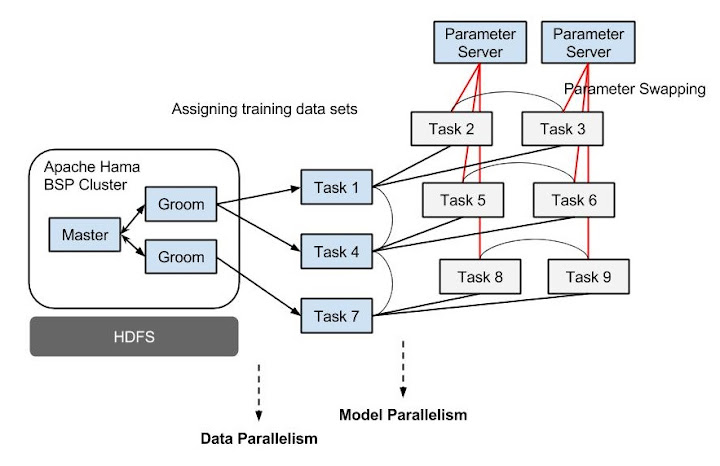Deep Learning has become a household buzzword these days. Google, Microsoft, and Tencent have developed distributed deep learning systems but, these systems are closed source softwares. Many of open source softwares such as DeepDist, Caffe, ..., etc are data parallel only. In this blog post, I introduce an Artificial Neural Network implementation of Apache Hama ML package and future design plan for supporting both data and model parallelism.

1. Artificial Neural Network of Hama ML Package

The lastest Apache Hama provides distributed training of an Artificial Neural Network using its BSP computing engine (the initial code was contributed by Yexi Jiang, a Hama committer, Facebook). In general, the training data is stored in HDFS and is distributed in multiple machines. In Hama, two kinds of components are involved in the training procedure: the master task and the groom task. The master task is in charge of merging the model updating information and sending model updating information to all the groom tasks. The groom tasks is in charge of calculate the weight updates according to the training data.

The training procedure is iterative and each iteration consists of two phases: update weights and merge update. In the update weights phase, each groom task would first update the local model according to the received message from the master task. Then they would compute the weight updates locally with assigned data partitions (mini-batch SGD) and finally send the updated weights to the master task. In the merge update phase, the master task would update the model according to the messages received from the groom tasks. Then it would distribute the updated model to all groom tasks. The two phases will repeat alternatively until the termination condition is met (reach a specified number of iterations).

The model is designed in a hierarchical way. The base class is more abstract than the derived class, so that the structure of the ANN model can be freely set by the user, as long as it is a layered model. Therefore, the Perceptron, Auto-encoder, Linear and Logistic regressor can all be uniformly represented by an ANN.

2. Future Plan for Large Scale Deep Neural Network

2.1 Architecture

As described in above section, currently the data parallelism is only used. Each node will have a copy of the model. In each iteration, the computation is conducted on each node and a final aggregation is conducted in one node. Then the updated model will be synchronized to each node. So, the performance is one thing; the parameters should fit into the memory of a single machine.

Here is a tentative near future plan I propose for applications needing large model with huge memory consumptions, moderate computational power for one mini-batch, and lots of training data. The main idea is use of Parameter Server to parallelize model creation and distribute training across machines. The basic idea of data and model parallelism is use of the remote parameter server to parallelize model creation and distribute training across machines, and the region barrier synchronization per task group instead of global barrier synchronization for performing asynchronous mini-batches within single BSP job. Each task group works asynchronously, and trains large-scale neural network model using assigned data sets in BSP paradigm. The below diagram shows an example of 3 task groups:Each task asynchronously asks the Parameter Server who stores the parameters in distributed machines for an updated copy of its model, computes the gradients on the assigned data, and sends updated gradients back to the parameter server. This architecture is inspired by Google's DistBelief (Jeff Dean et al, 2012).

2.2 Neuron-centric programming model

The new Programming API proposed by Edward J. Yoon will provide two user-defined functions, which the user can define the characteristic of artificial neural network model: Activation function and Cost function. Each function can be implemented by extending ActivationFunction and CostFunction abstract classes like below:

```  /**
* User-defined sigmoid actiavation function
*/
public static class Sigmoid extends ActivationFunction {
@Override
public double apply(double input) {
return 1.0 / (1 + Math.exp(-input));
}

@Override
public double applyDerivative(double input) {
return input * (1 - input);
}
}
```

The following properties are specified in the Job configuration:

• The model topology: including the number of neurons (besides the bias neuron) in each layer; the type of squashing function; the degree of parallelization for each layer.
• The learning rate: Specify how aggressive the model learning the training instances. A large value can accelerate the learning process but decrease the chance of model convergence. Recommend in range (0, 0.5].
• The momemtum weight: Similar to learning rate, a large momemtum weight can accelerate the learning process but decrease the chance of model convergence. Recommend in range (0, 0.5].
• The regularization weight: A large value can decrease the variance of the model but increase the bias at the same time. As this parameter is sensitive, it's better to set it as a very small value, say, 0.001.

The following is the sample design regarding how to create a job for training of three-layer neural network:

```  public static void main(String[] args) throws Exception {
ANNJob ann = new ANNJob();

// set learning rate and momentum weight
ann.setLearningRate(0.1);
ann.setMomentumWeight(0.1);

// initialize the topology of the model
// set the activation function and parallel degree.